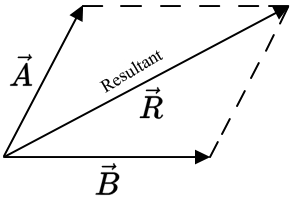## Friday, October 30, 2020

### Parallelogram Method for Vector Addition and Subtraction

The resultant of two vectors acting on any angle may be represented by the diagonal of a parallelogram. The two vectors are drawn as the sides of the parallelogram and the resultant is its diagonal, as shown below. The direction of the resultant is away from the origin of the two vectors.Each vector is resolved into its x-, y-, and z-components, with negatively directed components taken as negative. The scalar x-component, Rx, of resultant R is the algebraic sum of all the scalar components. The scalar y- and z-components of the resultant are found in a similar way. With components know, the magnitude for the resultant is given by

In two dimensions, the angle of the resultant with the x-axis can be found from the relation

Vector Subtraction

To subtract a vector B from a vector A, reverse the direction of B and add individually to vector A, that is